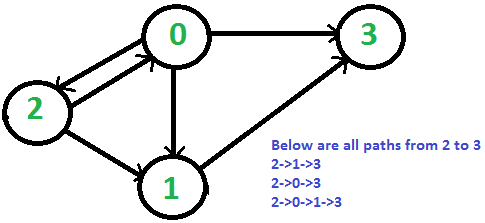# print all paths from a given source to a destination using BFS

Given a directed graph, a source vertex ‘src’ and a destination vertex ‘dst’, print all paths from given ‘src’ to ‘dst’.

Consider the following directed graph. Let the src be 2 and dst be 3. There are 3 different paths from 2 to 3.## Recommended: Please solve it on “PRACTICE” first, before moving on to the solution.

We have already discussed Print all paths from a given source to a destination using DFS.

Below is BFS based solution.

Algorithm :

create a queue which will store path(s) of type vector
initialise the queue with first path starting from src

Now run a loop till queue is not empty
get the frontmost path from queue
check if the lastnode of this path is destination
if true then print the path
run a loop for all the vertices connected to the
current vertex i.e. lastnode extracted from path
if the vertex is not visited in current path
a) create a new path from earlier path and
append this vertex
b) insert this new path to queue

 // CPP program to print all paths of source to  // destination in given graph  #include  using namespace std;     // utility function for printing  // the found path in graph  void printpath(vector<int>& path)  {      int size = path.size();      for (int i = 0; i < size; i++)           cout << path[i] << " ";          cout << endl;  }     // utility function to check if current  // vertex is already present in path  int isNotVisited(int x, vector<int>& path)  {      int size = path.size();      for (int i = 0; i < size; i++)           if (path[i] == x)               return 0;       return 1;  }     // utility function for finding paths in graph  // from source to destination  void findpaths(vector >&g, int src,                                    int dst, int v)  {      // create a queue which stores      // the paths      queue > q;         // path vector to store the current path      vector<int> path;      path.push_back(src);      q.push(path);      while (!q.empty()) {          path = q.front();          q.pop();          int last = path[path.size() - 1];             // if last vertex is the desired destination          // then print the path          if (last == dst)               printpath(path);                     // traverse to all the nodes connected to           // current vertex and push new path to queue          for (int i = 0; i < g[last].size(); i++) {              if (isNotVisited(g[last][i], path)) {                  vector<int> newpath(path);                  newpath.push_back(g[last][i]);                  q.push(newpath);              }          }      }  }     // driver program  int main()  {      vector > g;      // number of vertices      int v = 4;      g.resize(4);         // construct a graph      g.push_back(3);      g.push_back(1);      g.push_back(2);      g.push_back(3);      g.push_back(0);      g.push_back(1);         int src = 2, dst = 3;      cout << "path from src " << src           << " to dst " << dst << " are \n";         // function for finding the paths      findpaths(g, src, dst, v);         return 0;  }

Output:

path from src 2 to dst 3 are
2 0 3
2 1 3
2 0 1 3


This article is contributed by Mandeep Singh. If you like GeeksforGeeks and would like to contribute, you can also write an article using contribute.geeksforgeeks.org or mail your article to contribute@geeksforgeeks.org. See your article appearing on the GeeksforGeeks main page and help other Geeks.

posted @ 2020-02-09 16:50  chunchill  阅读(217)  评论(0编辑  收藏  举报